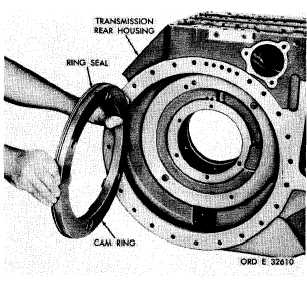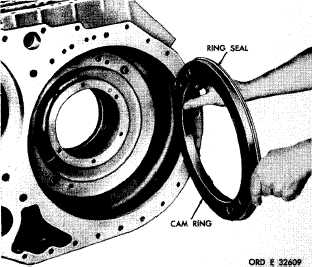Custom SearchPAR   1 5 6 R E A R    H O U S I N G    R E B U I LD C H A P    5,   SEC  X V I II Figure  190. Removing  (or  installing)  left-brake  apply cam stationary ring h. I n s t a l l    t h e    o u t p u t    d r i v e n    g e a r    w i t h bearing   inner   race   assemblies   into   tine   rear housing   (fig.   188). .   I f    b e a r i n g s    w e r e    r e m o v e d    f r o m    t h e o u t p u t     d r i v e     g e a r ,     i n s t a l l     a n     i n n e r - b e a r i ng race   assembly   on   each   side   of   the   gear   (fig. 1 8 7 ) .    P r e s s    b e a r i n g s    u n t i l    t h e y    a r e    f i r m ly seated   on   the   gear. j. Install   the   output   drive   gear   with   the b e a r i n g    i n n e r - r a c e    a s s e m b l i e s    i n t o    t h e    r e ar housing   (fig.   187). .   I f    b e a r i n g    o u t e r    r a c e s    w e r e    r e m o v e d from   supports   24   and   28   (fig.   377,   fold-out   6) and   34   and   2   (figs,   378   and   380,   fold-outs   7 and   9),   install   outer   bearing   races   in   the   sup- p o r t s. .   I n s t a l l    t h e    r i g h t - s t e e r    c l u t c h    s u p p o rt and   secure   with   five   3/8-16   self-locking   bolts ( f i g .    1 8 6 ) .    T o r q u e    t h e    b o l t s    t o    3 6 - 4 3    p o u n d - feet. m  .   I n s t a l l    t h e    r i g h t - o u t p u t    d r i v e    g e ar support   and   secure   with   five   3/8-16   self-lock- ing   bolts   (fig.   186).   Torque   the   bolts   to   36-43 p o u n d - f e e t . .   Install   the   left-output   drive   gear   sup- port   and   secure   with   five   3/8-16   self-locking Figure   191.   Removing   (or   installing)   right-brake   apply cam stationary ring b o l t s    ( f i g .    1 8 5 ) .    T o r q u e    t h e    b o l t s    t o    3 6 - 43 p o u n d - f e e t. o. I n s t a l l    t h e    l e f t - s t e e r    c l u t c h    s u p p o rt and   secure   with   five   3/8-16   self-locking   bolts (fig,   185).   Torque   bolts   to   36-43   pound-feet. .   If   bearings   78   and   80   (fig.   379,   fold- out   8)   were   removed   from   spindle   79,   install the    bearings,    pressing    against    the    numbered side   of   the   bearing   cage.   Press   each   bearing, 0.120   inch   below   the   end   of   the   spindle. .   I n s t a l l    t h e    l e f t - b r a k e    c a m    a s s e m b l y, spring   and   spindle   assembly   in   rear   housing. Install   the   spring   on   the   brake   cam   and   posi- tion   these   parts   in   the   housing,   then   install   the spindle   assembly   as   shown   (fig.   184). .   I n s t a l l    t h e    r e t a i n i n g    r i n g    a n d    s p a c er on   left-brake   apply   shaft   (fig.   183). .   I f    b e a r i n g    o r    o i l    s e a l    w a s    r e m o v e d, i n s t a l l    i n    r i g h t - b r a k e    a p p l y    s h a f t    ( f i g .    1 8 3 ). Install    bearing,    pressing    against    the    numbered side   of   the   cage,   0.960   inch   below   the   end   of the   shaft.   Install   the   new   replacement   oil   seal, l i p    s i d e    o f    s e a l    t o w a r d    t h e    i n s i d e ,    i n t o    t h e shaft   end   until   it   is   firmly   seated   against   its shoulder   in   the   shaft. .   Install   the   washer   and   retaining   ring on   the   right-brake   apply   shaft   (fig.   183). 1 3 5